﻿ 退役操盘手的告诫：A股唯一赚钱CCI指标，轻松抓获大牛股！_华股财经 ﻿

# 退役操盘手的告诫：A股唯一赚钱CCI指标，轻松抓获大牛股！

1、当CCI指标曲线在+100线～-100线的常态区间里运行时,CCI指标参考意义不大，可以用KDJ等其它技术指标进行研判。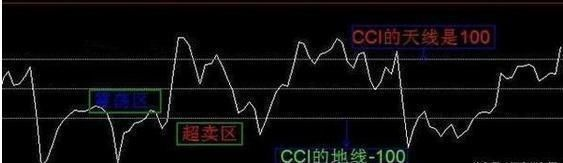2、CCI指标曲线从下向上突破+100线而进入非常态区间超买区后，当CCI指标曲线从上向下突破+100线而重新进入常态区间时，表明市场价格的上涨阶段可能结束，将进入一个比较长时间的震荡整理阶段，应及时平多做空。

3、当CCI指标曲线从上向下突破-100线而进入另一个非常态区间超卖区时，表明市场价格的弱势状态已经形成，将进入一个比较长的寻底过程，可以持有空单等待更高利润。如果CCI指标曲线在超卖区运行了相当长的一段时间后开始掉头向上，表明价格的短期底部初步探明，可以少量建仓。CCI指标曲线在超卖区运行的时间越长，确认短期的底部的准确度越高。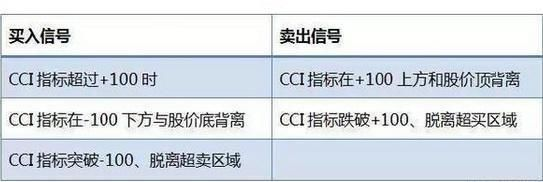4、CCI指标曲线从下向上突破-100线而重新进入常态区间时，表明市场价格的探底阶段可能结束，有可能进入一个盘整阶段，可以逢低少量做多。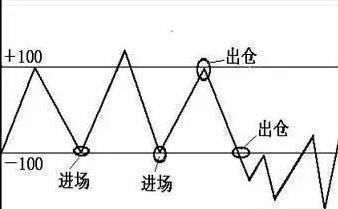CCI实战应用技巧

1.操盘线:即EXPMA，属于均线型的指数平均线指标。原参数为(12，50)，该参数属于中线参数，如果短线交易建议改为(7，21)。两条线的金叉作为买入的时机参考，特别是第一次金叉的成功率最高。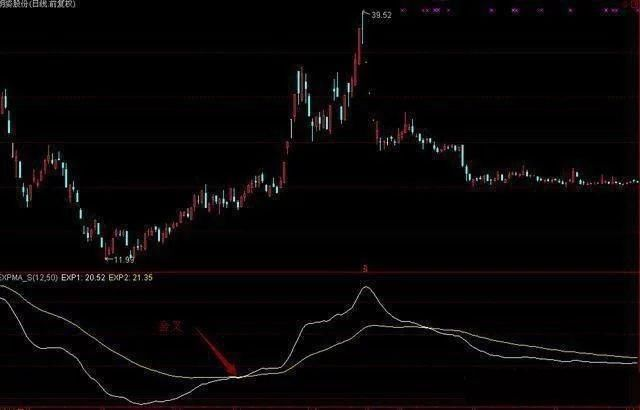2.底部与顶部的综合判断法:主要选取KDJ、CCI、BOLL、DMI四个指标结合判断。要求KDJ中J处于低位(或负值)、J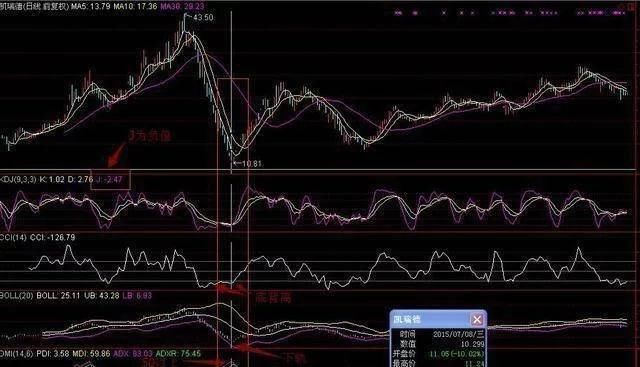3.大牛股的技术条件:5月线金叉10月线，则大牛股有望产生。所以请寻找5月线上的股票，一旦该股盘中踏上5日线(即不跌下超过30分钟)，即可买入。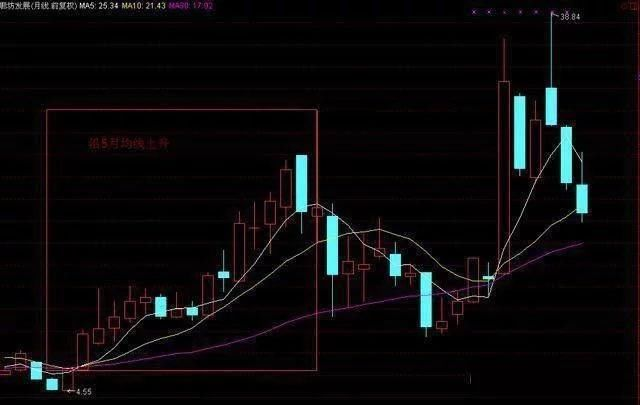X_1:=MA(CLOSE,9);

X_2:=POW(CLOSE-X_1,2);

X_3:=MA(X_2,20);

X_4:=SQRT(X_3);

X_5:=X_1+2*X_4;

X_6:=X_1-2*X_4;

X_7:=(CLOSE-LLV(LOW,9))/(HHV(HIGH,9)-LLV(LOW,9))*100;

X_8:=SMA(X_7,3,1);

X_9:=SMA(X_8,3,1);

X_10:=3*X_8-2*X_9;

X_11:=EMA(CLOSE,7)-EMA(CLOSE,13);

X_12:=EMA(X_11,5);

X_13:=(X_11-X_12)*2;

X_14:=REF(X_6,1);

CJ:LOWX_14 AND CLOSE>OPEN*1.03 AND X_10>=REF(X_10,1) AND X_13>=REF(X_13,1) AND VOL

﻿